HOME: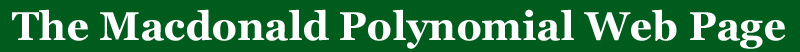Mark Haiman first announced a proof of the n!-conjecture at the 1999 CMS winter meeting in Montreal there were still gaps in this proof and he was fortunately able to complete it later. One announcement made by Adriano Garsia at the Winter Meeting in Montreal is that he is still offering a prize of \$1000 for the first mathematician who can produce bases for the n! modules.  Mark's result implies that such a basis exists but is not constructive in its nature.

# A Short Explanation of the n! Theorem

 For any partitionof n we define a polynomial in 2n variables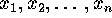andas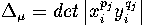where theare the coordinates of the cells of the partition when it is placed in the coordinate plane with the base cell at the position (0,0) and the rest of the coordinates have non-negative x and y values.

 Example: Consider the partition. The polynomialwill be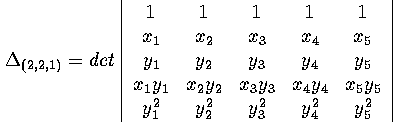Note that because this is a determinant, when an element of the symmetric group acts on this polynomial by permuting the x and the y variables simultaneously it permutes the columns, hence the action of the symmetric group on this polynomial is: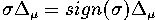Consider the linear span of all of the derivatives of this polynomial with respect to the variablesandand denote this space by. This vector space is closed under permutations that act on theand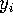simultaneously, and hence is a symmetric group module. The n! Theorem states that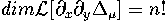It is a theorem by Garsia/Haiman that the dimension is less than or equal to n!

 Example: Because n! grows so quickly it is difficult to effectivly demonstrate an example of anything larger than 3. In this case we will choose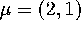.Consider all of the polynomials listed below, along with, they form a basis for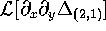(hence the dimension = 3! = 6)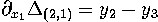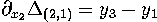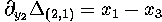The connection with the Macdonald polynomials comes when one considers the graded character of this module. Let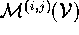be the character of the homogeneous component of of degree i in the x variables and degree j in the y variables of the polynomial vector space module. Consider the Frobenius image of the character of the n! module (that is,sends the irreducible representation indexed by the partition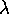to the Schur function indexed by the partition). Then define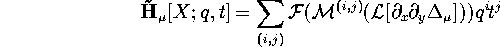A refined statement of the n! Conjecture is then that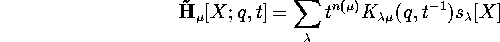whereare defined directly from the Macdonald polynomials.

 In our example above, if you are familiar with the representation of the symmetric group, it is not hard to see that the Frobenius image of the graded representation of this module is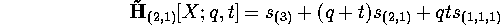since the polynomialis a copy of the alternating representation (of degree 1 in x and 1 in y), the polynomials of degree 1 in x and 0 in y (and similarly 0 in x and 1 in y) form a two dimensional representation corresponding to the partition (2,1), and the symmetric group acts on the constant terms with the trivial action (corresponding to the partition (3)).

This document was created with the program latex2html and then edited by hand on September 16, 1999.

The n! Conjecture was discovered by Adriano Garsia and Mark Haiman.
For a bibliography of the subject of the n! conjecture see the n! Conjecture Home Page.